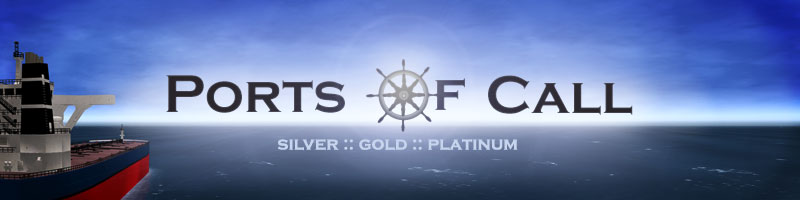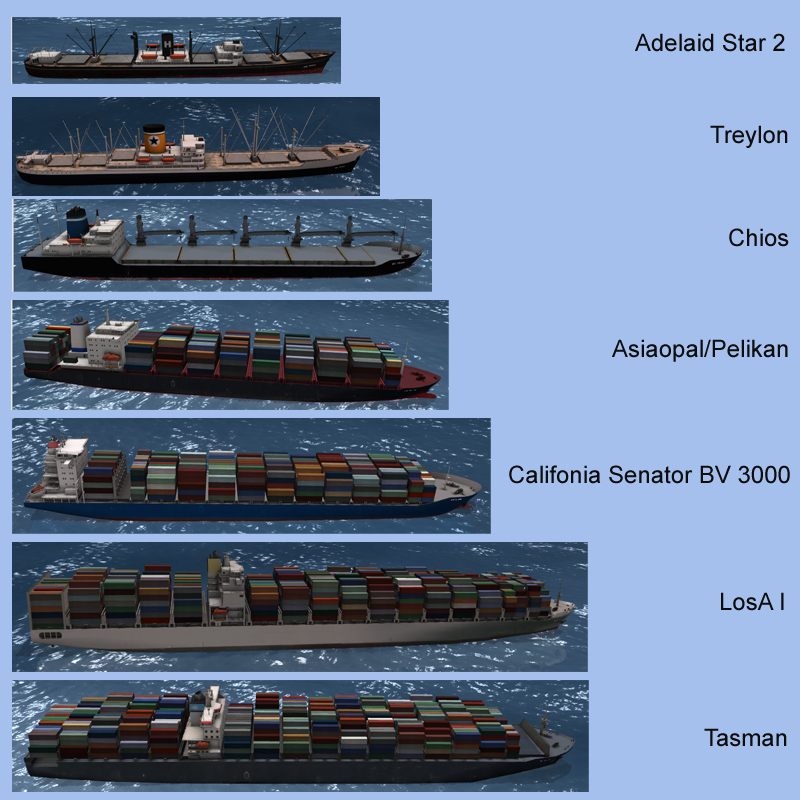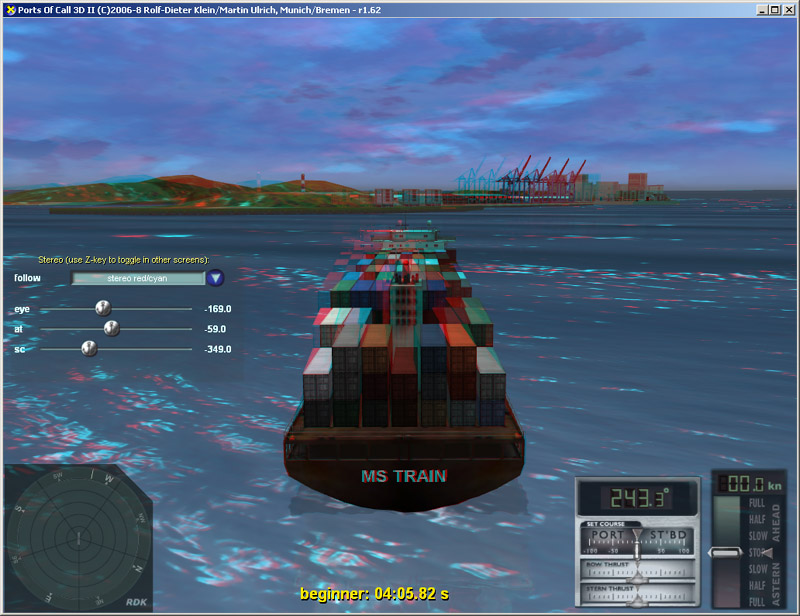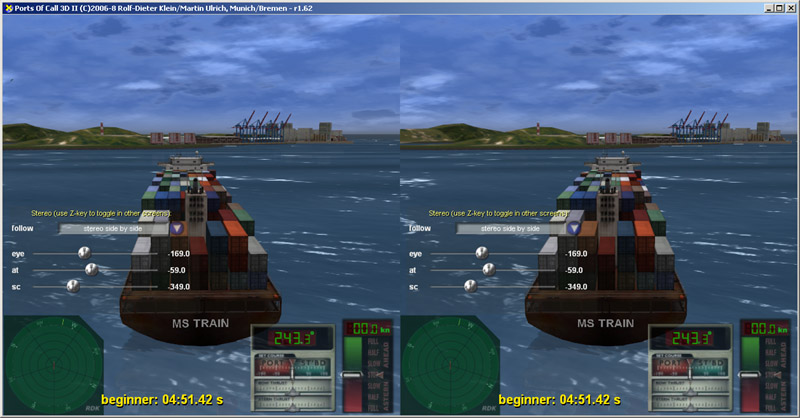## Expansions

we now have different expansion packs for Ports of Call. Some users made new ships, harbours and goods for POCXXL, available in our forum.
Here you can buy new expansion packs for POCSIM3DII, as new ships. And also a stereo license is available here for different stereo systems.

• POC SIM3DII expansion pack 1+2 new ships (attention: platinum license needed)
• POC SIM3DII stereo option (attention: platinum license needed)
• POC XXL for user addon look at the forum

 L Ports Of Call Expansion 1 €5,00 EUR Ports Of Call Expansion 2 €5,00 EUR Ports Of Call Stereo €19,00 EUR## Expansionpack 1

contained ships:
Hyndai Primorsky, length=139.97m width=21.50m GT=8689t teu=918 speed=15.00kn
Wan Hai 221. length=172.15m width=24.00m GT=16911t teu=168 speed=20.00kn
Bonn Express, length=235.65m width=32.30m GT=35919t teu=2803 speed=20.50kn
Zim Canada length=236.00m width=31.00m GT=37209t teu=2402 speed=21.00kn
Hamburg Express, length=320.58m width=42.80m GT=88493t teu=7506 speed=25.30kn
Xin Los Angeles, length=336.70m width=45.60m GT=108069t teu=9580 speed=25.20kn
Megapott, length=382.00m width=54.20m GT=185000t teu=13000 speed=26.00kn## Expansionpack 2

contained ships:
Treylon (Cargo), length=135.00m width=17.20m GT=5414t speed=13.00kn
Adelaid Star 2 (Cargo), length=175.00m width=22.20m GT=10000t speed=18.00kn
Chios (Cargo), length=180.80m width=26.00m GT=16177t speed=15.50kn
Asiaopal (=Pelikan), length=202.45m width=32.00m GT=30824t teu=1939 speed=17.00kn
Califonia Senator BV 3000, length=215.65m width=32.20m GT=34454t teu=3017 speed=15.00kn
LosA I, length=277.0m width=40.00m GT=66289t teu=5762 speed=24.90kn
Tasman, length=278.00m width=43.00m GT=78000t teu=5468 speed=25.50kn